Function Repository Resource:

# Primorial

Evaluate the primorial

Contributed by: Sander Huisman
 ResourceFunction["Primorial"][n] calculates the product of the primes up to n.

## Details

Integer mathematical function, suitable for both symbolic and numerical manipulation.
For n1, the primorial is equal to 1.
For n2, the primorial is defined as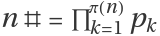, where pk is the kth prime and π(n) is PrimePi[n].
ResourceFunction["Primorial"] has the attribute Listable.

## Examples

### Basic Examples (2)

Compute the primorial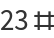:

 In:=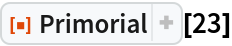Out=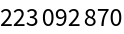Compute a list of the first 15 primorials:

 In:=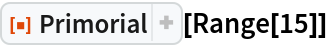Out=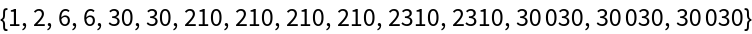### Scope (1)

For non-positive numbers, the primorial is defined to be equal to 1:

 In:=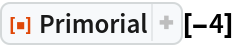Out=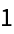### Properties and Relations (2)

Compare with the definition:

 In:=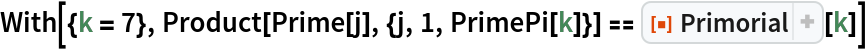Out=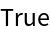The resource function ChebyshevTheta is the logarithm of the primorial:

 In:=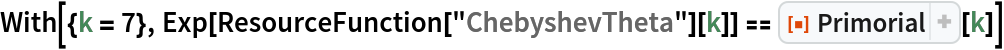Out=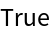### Neat Examples (3)

Evaluate the infinite primorial:

 In:=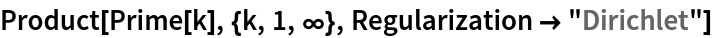Out=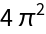In:=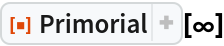Out=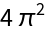Compare the growth rate of the primorial to that of the factorial:

 In:=Out=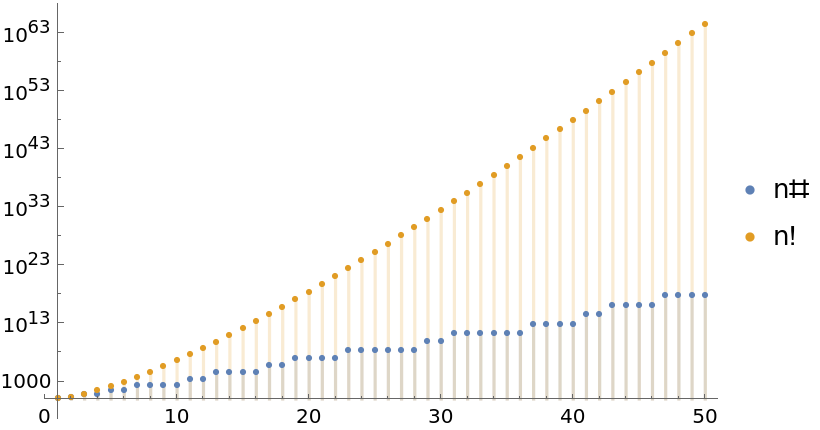Plot the differences between the factorial and the primorial up to n:

 In:=Out=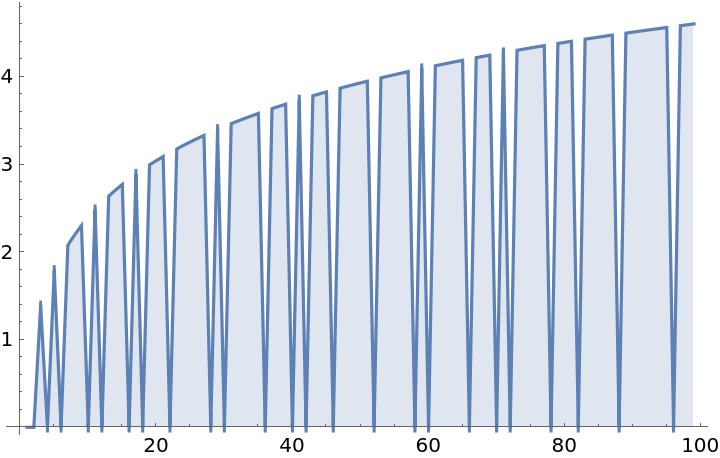SHuisman

## Version History

• 1.0.0 – 17 August 2021